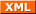# Christoph's last Weblog entries

### Entries from December 2013.

20th December 2013

Greetings from the FAU Security Team (FAUST), the Uni Erlangen CTF group. We were participating in the RuCTFe competition and made it to 4th place. Following is my write-up on the nsaless service, the main crypto challenge in the competition. nsaless is a nodejs webservice providing a short message service. People can post messages and their followers receive the message encrypted to their individual RSA key.

The gameserver created groups of 8 users on the service 7 were just following the first user (and authorized by the first user to do so) while the first user sent a tweet containing the flag. The service used 512bit RSA with 7 as public exponent. While RSA512 is certainly weak, it's strong enough to make it infeasible to break directly.

### Attacking RSA

There are some known attacks against RSA with small exponents if no proper padding is done. The most straightforward version just takes the e-th root of the cipher-text and, if the clear message was small enough, outputs that root as plain-text. As the flag was long enough to make this attack impossible, we need a somewhat improved Attack.

Reminder:

• In RSA, given a plain-text A, the sender computes Aᵉ mod N to build the cipher-text B.
• Given simultaneous congruences we can efficiently compute a x ∈ ℤ such that x satisfies all congruences using the Chinese remainder theorem.

For NSAless we actually get several such B for different N (each belonging to different users receiving the tweet because they follow the poster). This effectively means we get Aᵉ in mod N for different N. Using the Chinese remainder theorem we can now compute a x ∈ ℤ ≡ Aᵉ mod Π Nᵢ. If we use at least e different B for this we are guaranteed that x actually equals Aᵉ (in ℤ): A needs to be smaller than N for all N used (otherwise we lose information during encryption), therefore Aᵉ needs to be smaller than Nᵉ.

Computing now the e-th root of x we get the plain-text A – the flag.

### Fix

Fixing your service is easy enough, just increase e to an suitable number > 8. At the end of the contest 5 Teams had fixed this vulnerability by either using 17 or 65537.

### EXPLOIT

The basic exploit is shown below. Unfortunately it needs to retrieve all tweets for all users the compute the flags which just takes too long to be feasible (at least at the end of the competition where tons of users already existed) so you would need some caching to make it actually work. Would have been a great idea to have users expire after an hour or two in the service!

#!/usr/bin/python

import httplib
import urllib
import re
import json
import pprint
import gmpy
import sys

userparse_re = re.compile('<a [^>]*>([^<]*)</a></div>\s*<div>([^<]*)</div>')
tweetparse_re = re.compile("<div id='last_tweet'>([0-9]+)</div>")
followingparse_re = re.compile('<div><a href="/[0-9]+">([0-9]+)</a></div>')

def my_parse_number(number):
string = "%x" % number
if len(string) != 64:
return ""
erg = []
while string != '':
erg = erg + [chr(int(string[:2], 16))]
string = string[2:]
return ''.join(erg)

def extended_gcd(a, b):
x,y = 0, 1
lastx, lasty = 1, 0

while b:
a, (q, b) = b, divmod(a,b)
x, lastx = lastx-q*x, x
y, lasty = lasty-q*y, y

return (lastx, lasty, a)

def chinese_remainder_theorem(items):
N = 1
for a, n in items:
N *= n

result = 0
for a, n in items:
m = N/n
r, s, d = extended_gcd(n, m)
if d != 1:
raise "Input not pairwise co-prime"
result += a*s*m

return result % N, N

def get_tweet(uid):
try:
conn = httplib.HTTPConnection("%s:48879" % sys.argv, timeout=60)
conn.request("GET", "/%s" % uid)
r1 = conn.getresponse()
tweet = re.findall(tweetparse_re, data)
if len(tweet) != 1:
return None
followers = re.findall(followingparse_re, data)
return tweet, followers
except:
return None

def get_users():
conn = httplib.HTTPConnection("%s:48879" % sys.argv, timeout=60)
conn.request("GET", "/users")
r1 = conn.getresponse()
data = dict()
for i in re.findall(userparse_re, data1)[:100]:
userinfo = get_tweet(i)
if userinfo != None:

return data

users = get_users()
allusers = users.keys()
masters = [ user for user in allusers if len(users[user]) > 0 ]

for test in masters:
try:
followers = users[test]
data = []

for fol in followers:
n = int(users[fol])
tweet = int(users[fol])
data = data + [(tweet, n)]

x, n = chinese_remainder_theorem(data)

realnum = gmpy.mpz(x).root(7).digits()
print my_parse_number(int(realnum))
except:
pass

Tags: crypto, ctf, faust, ructf, security, writeup.Created by Chronicle v4.6